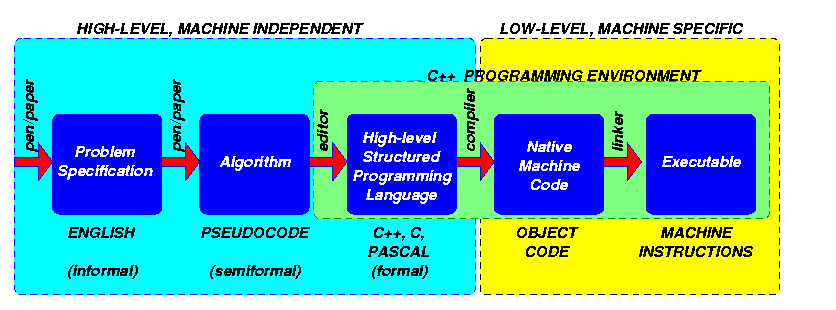Problem Specification Determine if a number is prime Algorithm input x for each number z that lies between 1 and x     if there is no remainder when z divides x then            output "not prime" and halt if no such number can be found then     output "prime" and halt High-level Structured Programming Language #include using namespace std; int main() {   int x;   cout << "enter number:" << endl;   cin >> x;   for (int z = 2; z

Back to the course